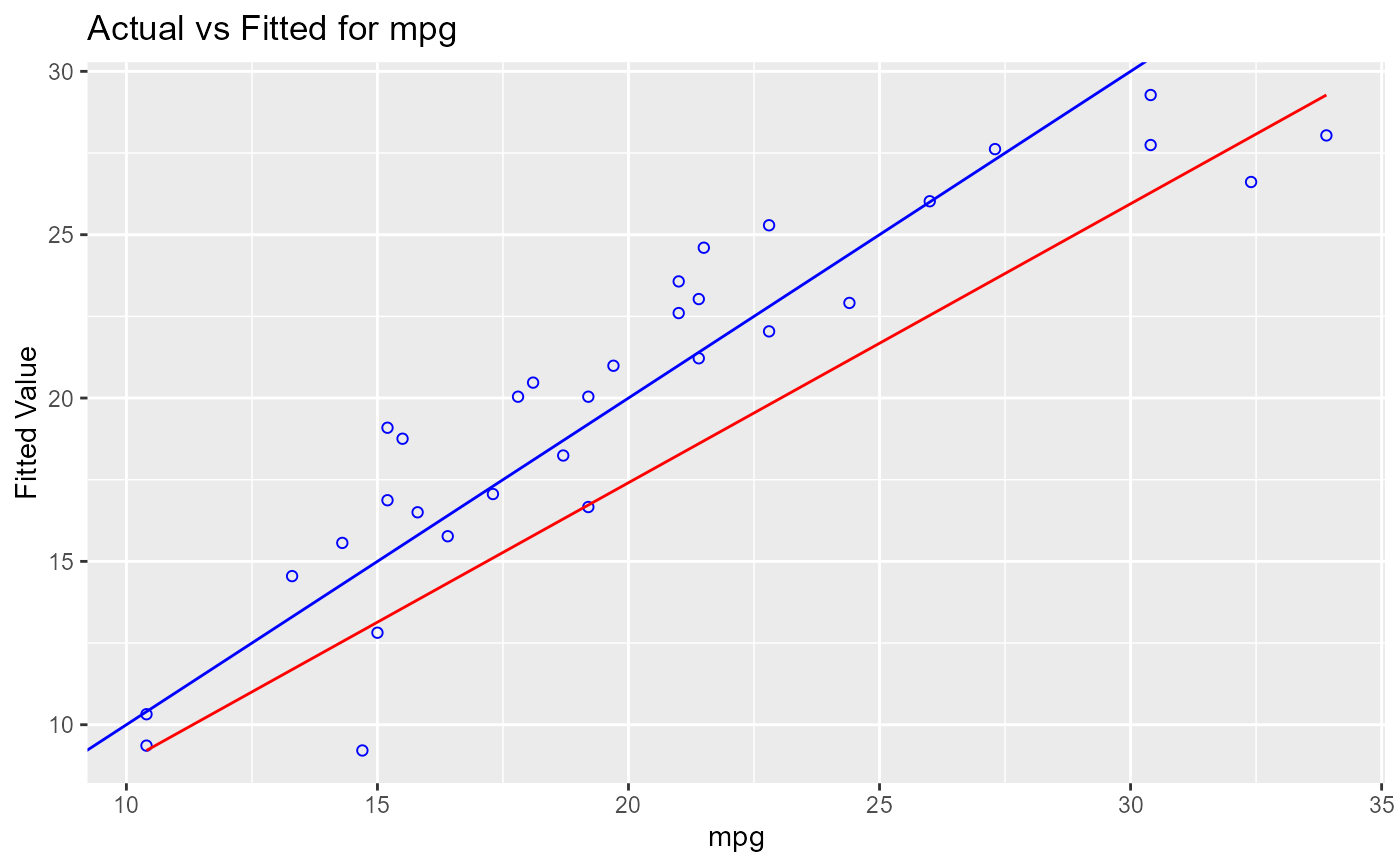Plot of observed vs fitted values to assess the fit of the model.

ols_plot_obs_fit(model, print_plot = TRUE)

## Arguments

model An object of class lm. logical; if TRUE, prints the plot else returns a plot object.

## Details

Ideally, all your points should be close to a regressed diagonal line. Draw such a diagonal line within your graph and check out where the points lie. If your model had a high R Square, all the points would be close to this diagonal line. The lower the R Square, the weaker the Goodness of fit of your model, the more foggy or dispersed your points are from this diagonal line.

## Deprecated Function

ols_ovsp_plot() has been deprecated. Instead use ols_plot_obs_fit().

## Examples

model <- lm(mpg ~ disp + hp + wt, data = mtcars)
ols_plot_obs_fit(model)